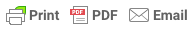Sorry, you do not have permission to ask a question, You must login to ask question. Become VIP Member

Do you need to remove the ads? Become VIP Member

The transfer of loads from a slab to beams is controlled by the slab’s geometrical dimension and the direction of reinforcements. The load of the slab, including self-weight, live load, and imposed dead load, are distributed over the beams on their sides.

The slab loads are expressed in weight per unit area, whereas the loads of beams are expressed in units of weight per length of the beam.

If the slab has regular dimensions, the load transfer can be carried out easily and quickly. However, if it has an irregular shape, it is recommended to use suitable programs such as SAP2000, SAFE, and ETABS.

## One-way Slab

The load of the one-way slab, which has a rectangular shape, is divided equally between adjacent beams. The interior beam takes half of the total load of a slab on each side.

If a slab is supported on two sides only or supported on all four sides, but the longer side to shorter side ratio is greater than 2, it is termed as one-way slab, see Figure-2.

## Two-way Slab

Loads on a two-way slab are transferred to all beams on all the sides. So, each beam supports an amount of the load from the slab. The slab is commonly divided into trapezoidal and triangular areas by drawing lines from each corner of the rectangle at 45 degrees.

The beam’s distributed load is computed by multiplying the segment area (trapezoidal or triangular area) by the slab’s unit load divided by the beam length. For an interior beam, the portion of the other side’s slab weight is estimated in a similar way and added to the previous one, i.e., the slab’s load from the other side of the beam. So, interior beams take loads from both the sides.

### Example

The slab of the figure shown below has a thickness of 150 mm and in addition to its own weight, it supports 0.85 KN/m2 partition, and a live load of 2.4 KN/m2. Transfer the load of the slab to the beams on all four sides.& Ad Free!

GET VIP MEMBERSHIP NOW!

### Solution:

Self-weight of the slab= slab thickness* concrete unit weight

= 0.15*24= 3.6 KN/m2

In this example, we use different load factors and then use load combination to calculate ultimate distributed load on the slab. After that, the ultimate distributed load is transferred to the beams.

Ultimate distributed load (Wu)= 1.2*4.45+1.4*2.4= 8.7 KN/m2

Load of slab on beam (4 m)= area of triangle*Wu

= 4*8.7=34.8 KN

Uniform distributed load of slab on beam (4 m) = 34.8/4= 8.7 KN/m

Load of slab on beam (4 m)= area of trapezoidal*Wu

= 8*8.7=69.6 KN

Uniform distributed load of slab on beam (6 m) = 69.6 /6= 11.6 KN/m

## Complex-geometry Slab

Finite element modeling should be used to distribute the load of a slab with complex geometry to a beam. For this purpose, computer programs like SAP200, SAFE, and ETABS can be used. This method can also be considered for slabs with regular geometry.

## FAQs

How is load transferred from slab to beams?

In a one-way slab, loads are transferred in only one direction, whereas the loads on a two-way slab are transferred in two directions.

What are the main types of loads on structures?

How is the live load on slab calculated?

The live load on a slab is determined based on the function of the structure. For instance, use 2.4 KN/m2 (50 psf) for offices, as per Table 4-1 in ASCE standard (ASCE/SEI 10-7).

The dead load of a concrete member is calculated by multiplying the volume of the concrete element by concrete unit weight.

What is imposed load on a building?

Imposed load is described as a load that is applied to a structure and is not permanent during the life service of the structure and may vary.

How Stresses are Transferred from R.C. Columns to Footings?

Types of Loads on Structures – Buildings and Other Structures